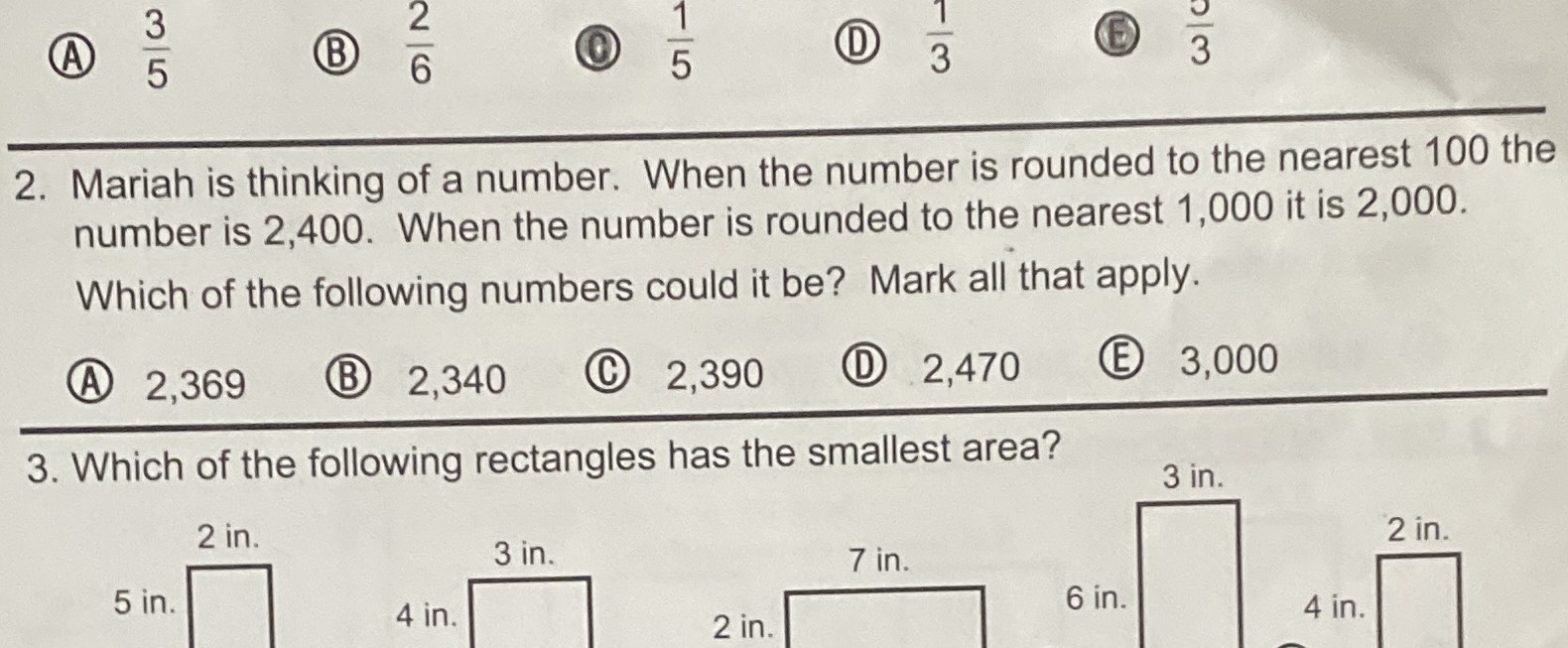### ¿Todavía tienes preguntas de matemáticas?

Pregunte a nuestros tutores expertos
Algebra
Pregunta2. Mariah is thinking of a number. When the number is rounded to the nearest $$100$$ the number is $$2,400$$ . When the number is rounded to the nearest $$1,000$$ it is $$2,000$$ . Which of the following numbers could it be? Mark all that apply. (A) $$2,369$$ (B) $$2,340$$ (C) $$2,390$$ (D) $$2,470$$ (E) $$3,000$$